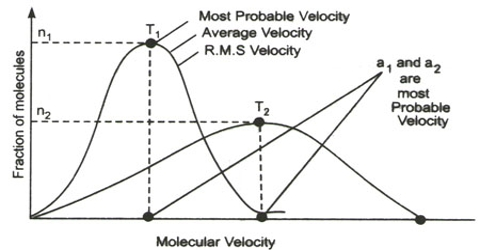# Molecular Velocities

Molecular Velocities

The kinetic equation [PV = 1/3 mnc2] may be used directly for calculating the r.m.s velocity of a molecule of any gas. One can rearrange equation (PV = 1/3 mnc2) as,

c = √(3PV/mn)

For one mole of gas mn = M, so that,

c = √(3PV/M) = √(3RT/M) … … … (since PV=RT)

Example: Calculator the r.m.s. velocity of hydrogen gas (RMM = 2.016) at 250C

Solution:

The r.m.s. velocity of a hydrogen molecule is given by;

c = √(3RT/M) = √[(3×8.314×107x298)/2.016] cm s-1

= 1.92 x 105 cm s-1

Any of the following forms of the Kinetic Equation may be used for calculating molecular velocities:

c = √(3PV/M) = √(3P/ρ) = √(3RT/M)

It must, however, be remembered that if the velocity is to be obtained in the c.g.s units. i e., in centimeter per second, all if quantities P, V, ρ, M and R have to be expressed in c.g.s. units.

As may be seen from above, the velocity or hydrogen molecular at ordinary temperature is very high — more than s mile of second. The velocity is inversely proportional to the square root of the molecular mass heavier molecules like those of carbon dioxide or uranium hexafluoride will have lower velocity than that of hydrogen.